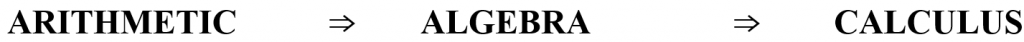# Book VII

We study:

• Geometry
• Trigonometry
• Measurement
• Circles
• Arc length, Angular Speed & Linear Speed
• Revolutions Per Minute (RPM)
• Measurement – Area of a circle
• The Trigonometric Ratios
• Extension of Right Triangle Trigonometry to xy-plane
• Basic Trigonometric identities
• Applications of Trigonometric Ratios
• Inverse Trigonometric Ratios
• Law of Sines & Cosines
• ARITHMETIC – ALGEBRA – CALCULUS
• Understanding Rate of Change
• Instantaneous Rate of Change
• Relations and Functions
• What is a Function?
• Domain and Range
• Applications of Functions

As shown we explore the history of Arithmetic, Algebra, & Trigonometry and the transition to Calculus.

The “MATH YES WE CAN” program is designed to help you learn basic Mathematical concepts that will enable you to master the ideas that form the foundation of mathematics and make a successful TRANSITION to CALCULUS